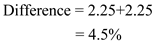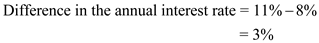# Quiz 21: Optimum Currency Areas and the Europage 669 How the European Single Currency Evolved

According to the interest parity condition of the foreign-exchange market equilibrium, there should be a positive relationship between the interest rate differential and the exchange rate. Interest rate differential is calculated by deducting foreign country interest rate from the home country's interest rate. The exchange rate is the price of the foreign country's currency in terms of the home country currency. Since the exchange rate between the Italian and the Germany currency that is lira/DM exchange rate fluctuates within the band of ±2.25, the maximum difference between the interest rates on one-year lira and DM deposits is:Now, for six months lira and DM deposits, the interest will compound twice a year. So, the maximum difference between the interest rates over six months is:Similarly, for three months lira and DM deposits, the interest will compound four times a year. So, the maximum difference between the interest rates over three months is:The answers are different in each case because, the interest rates are calculated on compounding basis.
Given that: Annual interest rate on five-year government bond in Italy is 11%. Annual interest rate on five-year government bond in Germany is 8%. First, calculate the difference in the annual interest of Italy and Germany:Now, calculate the rate if there is 3% difference over five year's bond:So, the expected 15.9% change in the lira/DM exchange rate over five years is much above the EMS currency band of ±2.25. Therefore, with 3% interest differentials, there is little credibility for maintaining current lira/DM exchange rate parity.